• 图像的几何变换是将一幅图像的坐标映射到另外一幅图像的新坐标位置，它不改变图像的像素值，只是改变像素所在的几何位置，使原始图像按照需要产生位置、形状和大小的变换。 1、图像的平移 2、图像的镜像 3、图像...
图像的几何变换
图像的几何变换是将一幅图像中的坐标映射到另外一幅图像中的新坐标位置，它不改变图像的像素值，只是改变像素所在的几何位置，使原始图像按照需要产生位置、形状和大小的变换。
1、图像的平移
在MATLAB中，没有提供具体图像平移函数，直接运用MATLAB指令编程即可实现图像的平移操作。
MATLAB实现
示例：
构造图像平移函数
function [output_image] = move(input_image,m,n)
%定义move函数，input_image为输入图像，m为输入图像沿着水平方向移动的距离，n为输入图像沿着垂直方向移动的距离，output_image为平移后的输出图像
%不考虑图像平移以后的溢出情况，找不到对应点的地方都赋值为1
[M,N,G]=size(input_image);    %获取输入图像的大小
double_image=im2double(input_image);   %将数据图像类型转换成双精度
new_image=ones(M,N,G);    %初始化新图像矩阵全为1，大小与输入图像相同
for i=1:M
for j=1:N
if((i+m)>=1&&(i+m)<=M&&(j+n)>=1&&(j+n)<=N)      %判断平移以后行列坐标是否超出范围
new_image(i+m,j+n,:)=double_image(i,j,:);    %进行图像平移
end
end
end
output_image=new_image;

调用函数，实现图像平移
clear all; close all; clc;
input=imread('G:\picture\Fig0450(a)(woman_original).tif');
output1=move(input,100,100);
output2=move(input,-100,-100);
subplot(1,3,1); imshow(input); title('原图像');
subplot(1,3,2); imshow(output1); title('右下平移后的图像');
subplot(1,3,3); imshow(output2); title('左上平移后的图像');

运行结果：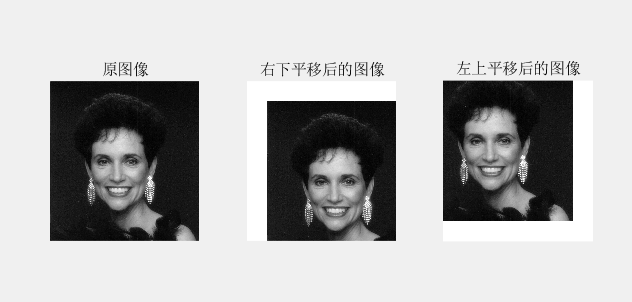2、图像的镜像
图像的镜像分为垂直镜像和水平镜像。
MATLAB实现
示例：
构造图像镜像函数
function [outImage] = mirror(InImage,n)
%定义mirror函数实现图像镜像变换
%参数n为1时，实现水平镜像变换
%参数n为2时，实现垂直镜像变换
%参数n为3时，实现水平垂直镜像变换
[M,N,G]=size(InImage); %获取输入图像InImage的大小
transImage=InImage;
if n==1
for i=1:M
for j=1:N
transImage(i,M+1-j,:)=InImage(i,j,:);
end
end
elseif n==2
for i=1:M
for j=1:N
transImage(M+1-i,j,:)=InImage(i,j,:);
end
end
elseif n==3
for i=1:M
for j=1:N
transImage(M+1-i,M+1-j,:)=InImage(i,j,:);
end
end
else
error('参数n输入不正确，n取1、2、3');
end
outImage=transImage;

调用函数，实现图像镜像
clear all; close all; clc;
input=imread('G:\picture\Fig0450(a)(woman_original).tif');
output1=mirror(input,1);
output2=mirror(input,2);
output3=mirror(input,3);
subplot(2,2,1); imshow(input); title('原图像');
subplot(2,2,2); imshow(output1); title('水平镜像');
subplot(2,2,3); imshow(output2); title('垂直镜像');
subplot(2,2,4); imshow(output3); title('水平垂直镜像');

运行结果：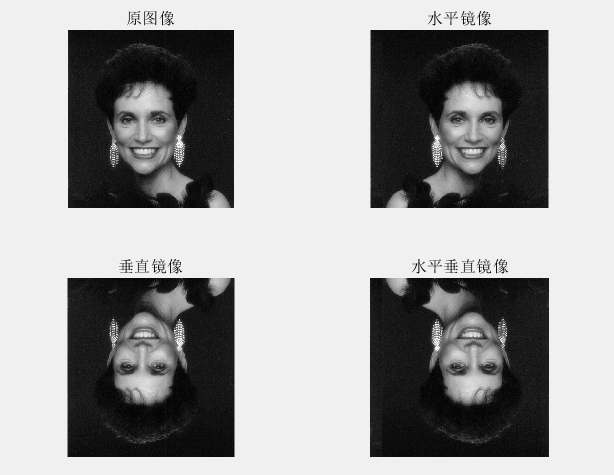3、图像的缩放
图像的缩放是指将给定的图像在x轴方向按比例缩放fx倍，在y轴方向按比例缩放fy倍，从而获得一幅新的图像。如果fx=fy，即在x轴方向和y轴方向缩放的比例相同，即为图像的全比例缩放。如果fx≠fy，图像比例缩放会改变原始图像像素间的相对位置，产生几何畸变。
–imresize()函数
MATLAB实现
示例：
clear all; close all; clc;
InImage=imread('G:\picture\Fig0450(a)(woman_original).tif');
shrink1=imresize(InImage,0.5);  %设置缩放比例，缩小图像
enlarge1=imresize(InImage,2);  %设置缩放比例，扩大图像
shrink2=imresize(InImage,[500 600]);  %设置缩放后的图像行列，实现缩放图像并显示
shrink3=imresize(InImage,[NaN 600]);  %函数按照输入图像纵横比生成行数，实现缩放图像并显示
enlarge2=imresize(InImage,1.5,'bilinear');  %采用双线性插值法对图像进行缩放
enlarge3=imresize(InImage,1.5,'triangle');  %采用三角型核函数插值对图像进行缩放

subplot(2,4,1); imshow(InImage); title('原图像');
subplot(2,4,2); imshow(shrink1); title('shrink1');
subplot(2,4,3); imshow(enlarge1); title('enlarge1');
subplot(2,4,4); imshow(shrink2); title('shrink2');
subplot(2,4,5); imshow(shrink3); title('shrink3');
subplot(2,4,6); imshow(enlarge2); title('enlarge2');
subplot(2,4,7); imshow(enlarge3); title('enlarge3');

运算结果：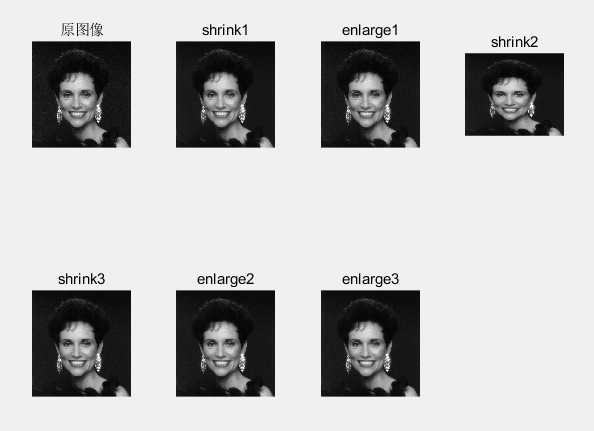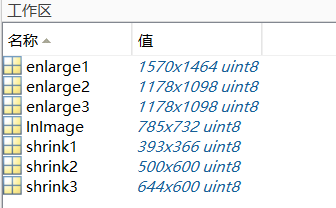4、图像的转置
图像转置即为图像的行列坐标互换，进行图像转置后，图像的大小会发生变化。
MATLAB实现
示例：
function [outputImage] = transposition(inputImage)
% inputImage为输入图像
% outputImage为对输入图像转置的输出图像
[M,N,G]=size(inputImage);
inputImage=im2double(inputImage);
transp=ones(N,M,G);
for i=1:M
for j=1:N
transp(j,i,:)=inputImage(i,j,:);
end
end
outputImage=transp;
end

运行结果：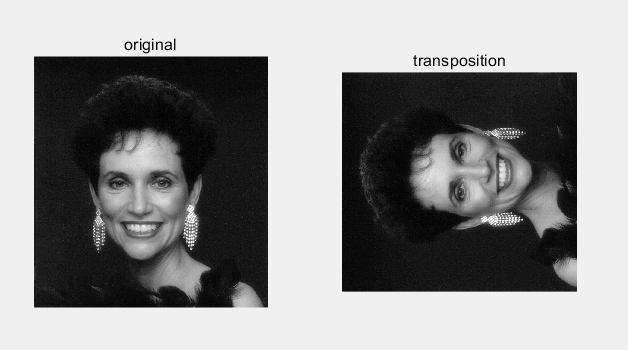5、图像的旋转
图像的旋转变换属于图像的位置变换，通常是以图像的中心为原点，将图像上的所有像素都旋转一个相同的角度。旋转后，图像的大小一般会改变。
–imrotate()函数
MATLAB实现
示例：
clear all; close all; clc;
InImage=imread('G:\picture\Fig0227(a)(washington_infrared).tif');
rotate1=imrotate(InImage,20); %将图像以其中心为原点逆时针旋转20°，采用最近邻插值生成完整旋转图像
rotate2=imrotate(InImage,-20); %将图像以其中心为原点顺时针旋转20°，采用最近邻插值生成完整旋转图像
rotate3=imrotate(InImage,20,'bilinear'); %将图像以其中心为原点逆时针旋转20°，采用双线性插值生成完整旋转图像
rotate4=imrotate(InImage,20,'bilinear','crop'); %将图像以其中心为原点逆时针旋转20°，采用最近邻插值,对旋转后图像裁剪保证输出图像与输入图像大小相等
rotate5=imrotate(InImage,20,'bilinear','loose'); %将图像以其中心为原点逆时针旋转20°，采用最近邻插值生成完整旋转图像

subplot(2,3,1); imshow(InImage);
subplot(2,3,2); imshow(rotate1);
subplot(2,3,3); imshow(rotate2);
subplot(2,3,4); imshow(rotate3);
subplot(2,3,5); imshow(rotate4);
subplot(2,3,6); imshow(rotate5);

运行结果：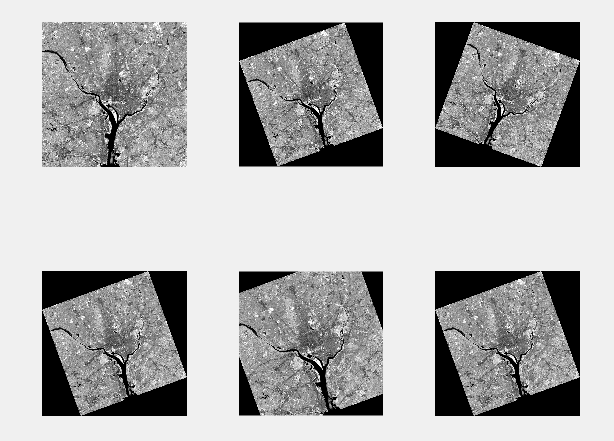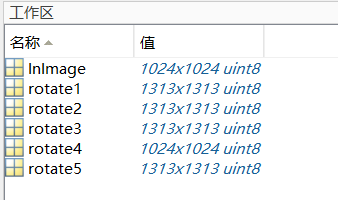6、图像的剪切
–imcrop()函数
MATLAB实现
示例：
clear all; close all; clc;
InImage=imread('G:\picture\Fig0227(a)(washington_infrared).tif');
rect=[445 625 130 200]; %定义剪切区域
X=imcrop(InImage,rect); %进行图像剪切
subplot(1,2,1); imshow(InImage); title('原图像');
rectangle('Position',rect,'LineWidth',2,'EdgeColor','r'); %将图像的剪切区域标出
subplot(1,2,2); imshow(X); title('剪切图像');

运行结果：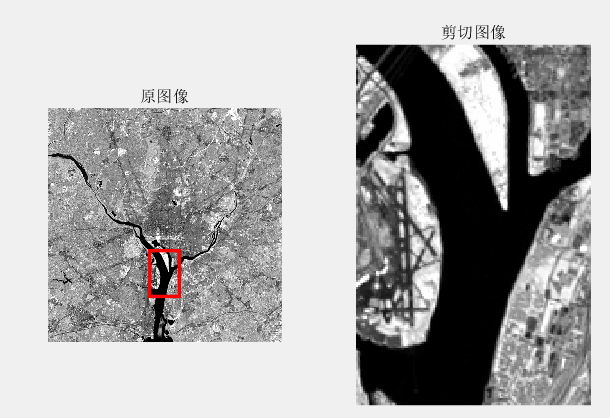7、图像的空间变换
在MATLAB的图像处理工具箱中提供了一个专门的函数 intransform（），可以通过定义参数实现多种类型的空间变换，包括仿射变换（如平移、缩放、旋转、剪切）、投影变换等。


展开全文• MATLAB在数字图像处理有很多几何变换的函数，以下为基本例子 首先读取原图像再一次进行几何变换 P=imread('picture1.png'); 1、图像旋转 imrotate(picture，angle，‘bilinear’or’nearest’); q其中picture是...
一、基本介绍与应用
MATLAB在数字图像处理中有很多几何变换的函数，以下为基本例子
首先读取原图像再一次进行几何变换
P=imread('picture1.png');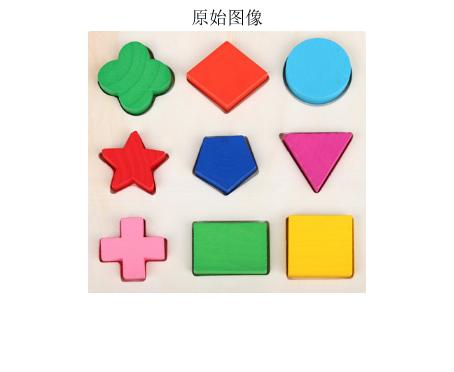1、图像旋转
imrotate(picture，angle，‘bilinear’or’nearest’);
q其中picture是图像储存的矩阵，angle为旋转角度，顺时针为负，逆时针为正
p_rotate=imrotate(P,-20,'bilinear');        %双线性插值法顺时针旋转20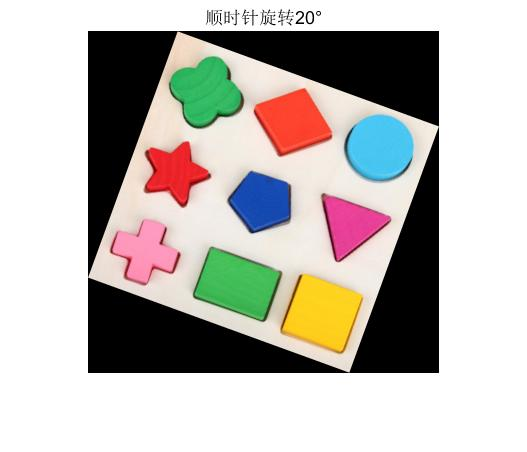2、图像镜像
fliptr(picture);  //左右镜像
fliput(picture);//上下镜像
flipdim(picture,DIM);//按照特定轴翻转，1为行翻转，2为列翻转
p_Hzmirror=fliplr(p_rotate);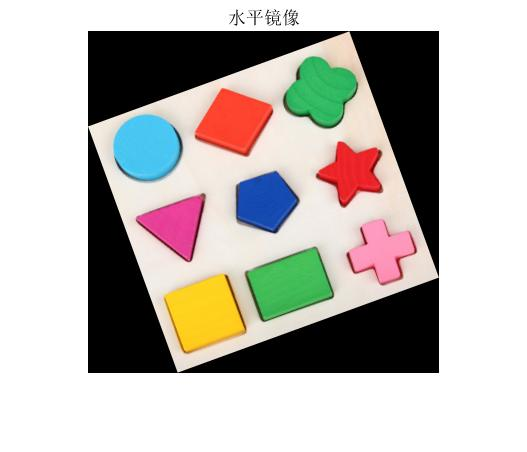3、图像错切
tform1=maketform('affine',[1 0.5 0;0.3 1 0;0 0 1]);     %kx=0.3,ky+0.5错切
p_trans3=imtransform(im2double(P),tform1,'bilinear');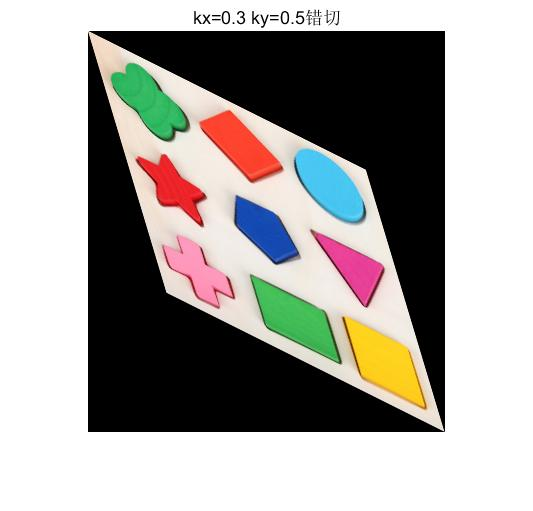4、图像缩小或者放大
p_size=imresize(p_trans3,0.1,'bilinear');//kx=ky=0.1缩小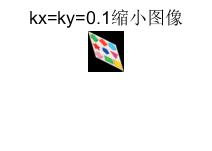二、利用几何变换和图像的代数运算，将两幅图像生成一副图像
……待续


展开全文• 学习图像中的仿射变换(affine transform), 这是一种线性变换（涵盖旋转，平移，错切(shear), 缩放等线性变换），既然是线性变换则可以通过线性变换（矩阵）来获得。仿射变换矩阵M为2*3矩阵。仿射变换的性质有...
   学习图像中的仿射变换(affine transform), 这是一种线性变换（涵盖旋转，平移，错切(shear), 缩放等线性变换），既然是线性变换则可以通过线性变换（矩阵）来获得。仿射变换矩阵M为2*3的矩阵。仿射变换的性质有“平直性”和“平行性”。即直线经过affine以后还是直线，两条平行的线经过affine以后依旧是平行的。 透视变换(prespective transform),也是一种线性变换，只不过其中3*3的矩阵来表达，其拥有8个自由度。其数学原理和affine_transform是一样的。 表达式为：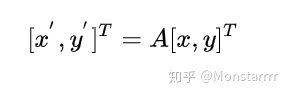其中表示变换后的坐标， [x,y]表示原始坐标。所以一般的逻辑步骤为，首先我们获取src ---> dst的变换矩阵，然后将这个矩阵应用到原始图像上去。但是实际中这样的做是有风险的。首先所面临的风险是采样失真，原因是因为经过变换后原始图像的整数像素点可能变为小数像素点。这涉及到插值的部分，但是因为有可能目标图像上该点周围并无整数点像素而无法使用周围四个点进行双线性插值，第二个风险就是原始图像上的某些点经过映射后超出了图像的画幅，这时需要策略来处理这种情况。所以我们可以这样做，求dst ---> src的变换，即通过目标图像的像素来求原始图像上对应点。这里其实需要处理两个问题：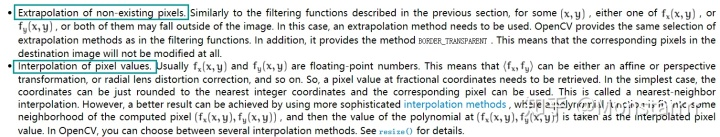参考信息来自opencv官方文档 即外推原始图像上不存在的像素点和插值有小数点位置的像素值。opencv中有cv2.getAffineTransform(src, dst)方法来获取仿射矩阵。这里面src和dst表示对应的三个非共线点的坐标以及目标图像对应的三个点的坐标。（为什么需要三个点？因为这里涉及到解线性方程问题，而仿射矩阵有6个自由度，那么至少需要三个点就能解出方程了，为自由度的一半。这个最简单的方法是x+y=1, 两个自由度，给出x就能求出y了）。获得仿射矩阵以后，使用cv2.warpAffine(img, affine_matrix, dsize).这表面上是从src-->dst的过程。但是实际上计算的时候是计算从dst --> src （可以用cv2.invertAffineTransform(affine_matrix)将仿射矩阵的逆操作计算出来），这样的话每次就是从dst对应的坐标中寻找src所对应的像素点（也许是整数点，也许的带小数点的，也是不在src的图像范围内）。整数点就直接copy， 小数点就直接双线性插值，超出边界了要么外推该像素点的像素要么就不改变该点的像素值。说完了opencv是怎么做的，那么来看看torch中的是怎么实现这个的。Torch.nn.functional 中也有实现类似变换的过程。但是这里面就直接是从dst --> src。所以输入的仿射矩阵就应该是dst --> src。 而不是像opencv中从src ---> dst 这样来输入仿射矩阵。这里面需要两个函数配合使用。grid = affine_grid(invert_affine_matrix, input_size), 获取输出的每个点对应与输入的索引。 然后使用grid_sample(image, grid)得到最终的输出图像。里面原理我怀疑和opencv的类似。有了上面的讲解我们就不难理解这个仿射矩阵所作的事是对原始图像方法一倍。（原始仿射矩阵[[2, 0, 0], [0, 2, 0]], 逆仿射矩阵为[[0.5, 0, 0], [0, 0.5, 0]]）参考资料： 【pytorch affine_grid的用法】 【opencv 几何变换】 【图像中的坐标变换(刚体变换、相似变换、仿射变换、投影变换)】 【Spatial Transformer Networks 详细解析】（STN网络的本质就是学习一个仿射变换矩阵，使得 图像/特征 应用这个仿射矩阵后可以将一些例如旋转，缩放，平移等形变给cover到，因此使得网络得到了cover形变的能力，但是因为对于形变处理这部分是没有监督信息的，所以整个的形变效果是严重依赖数据集的形变分布的） 知识延伸：将STN模块组合目标检测中去 ？ 未完待续。
展开全文• 介绍上面的图像使它不言而喻什么是几何变换。它是一种应用广泛的图像处理技术。例如，在计算机图形学有一个简单用例，用于在较小或较大屏幕上显示图形内容时简单地重新缩放图形内容。它也可以应用于扭曲一个...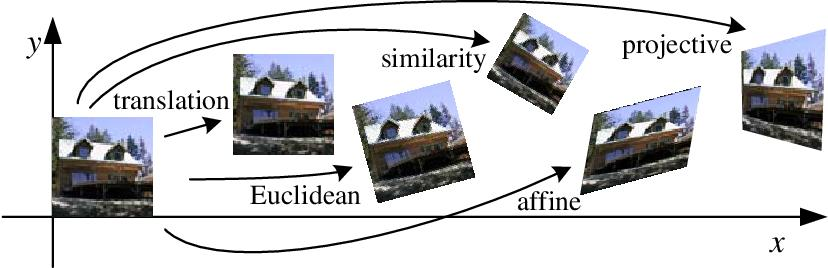介绍上面的图像使它不言而喻什么是几何变换。它是一种应用广泛的图像处理技术。例如，在计算机图形学中有一个简单的用例，用于在较小或较大的屏幕上显示图形内容时简单地重新缩放图形内容。它也可以应用于扭曲一个图像到另一个图像平面。例如，与其直视前方的场景，不如自上而下地看。在这个场景中应用透视图变换来实现这一点。另一个应用是训练深层神经网络。训练深度模型需要大量的数据。在几乎所有的情况下，模型都受益于更高的泛化性能，因为有更多的训练图像。人工生成更多数据的一种方法是对输入数据随机应用仿射变换(增强)。在本文中，我将向你介绍一些变换，以及如何在Numpy和OpenCV中执行这些变换。特别是，我将关注二维仿射变换。你需要的是一些基本的线性代数知识。仿射变换的类型在不涉及太多数学细节的情况下，变换的行为由仿射A中的一些参数控制。x’ = Ax其中A是在齐次坐标系中的2x3矩阵或3x3，x是在齐次坐标系中的(x，y)或(x，y，1)形式的向量。这个公式表示A将任意向量x，映射到另一个向量x’。一般来说，仿射变换有6个自由度。根据参数的值，它将在矩阵乘法后扭曲任何图像。变换后的图像保留了原始图像中的平行直线(考虑剪切)。本质上，满足这两个条件的任何变换都是仿射的。但是，有一些特殊形式的A，这是我们将要讨论的。这包括旋转、平移和缩放矩阵，如下图所示。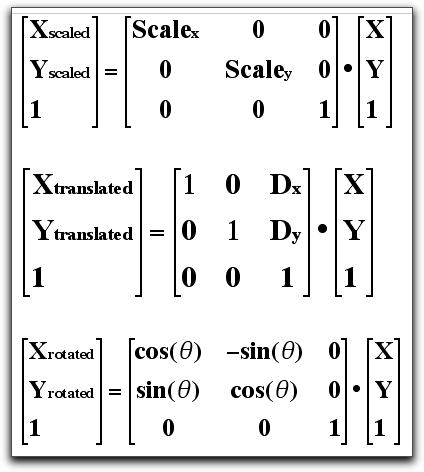上述仿射变换的一个非常有用的性质是它们是线性函数。它们保留了乘法和加法运算，并遵循叠加原理。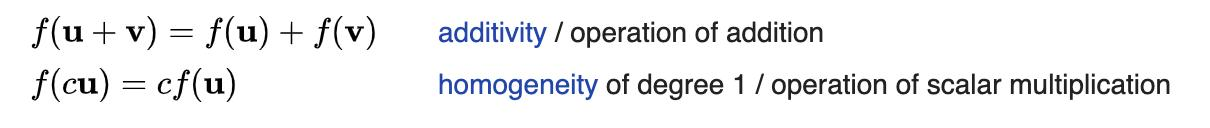换言之，我们可以组合2个或更多的变换：向量加法表示平移，矩阵乘法表示线性映射，只要我们用齐次坐标表示它们。例如，我们可以将旋转和平移表示为A = array([[cos(angle),  -sin(angle), tx],            [sin(angle), cos(angle),  ty],            [0,          0,           1]])图像表示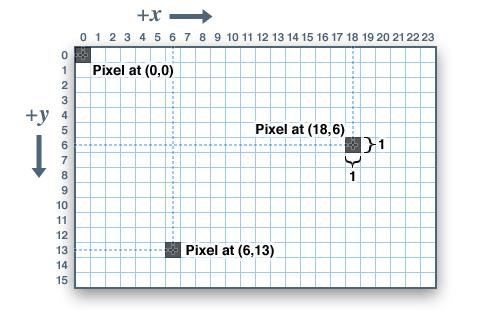在Python和OpenCV中，2D矩阵的原点位于左上角，从x，y=(0，0)开始。坐标系是左手的，X轴指向右，Y轴指向正下方。但在教科书和文献中，如上面所示的3个矩阵，大多数变换矩阵都遵循右手坐标系。因此，必须进行一些小的调整来调整轴线方向。欧氏空间中的公共变换在我们对图像进行变换实验之前，让我们看看如何在点坐标上进行变换。因为它们本质上与图像是网格中的二维坐标数组相同。利用上面的知识，下面的代码可以用来变换(0,0)，(0,1)，(1,0)，(1,1)处的点。此外，Python还提供了一个有用的速记运算符@来表示矩阵乘法。# 点生成器def get_grid(x, y, homogenous=False):    coords = np.indices((x, y)).reshape(2, -1)    return np.vstack((coords, np.ones(coords.shape))) if homogenous else coords# 定义变换def get_rotation(angle):    angle = np.radians(angle)    return np.array([        [np.cos(angle), -np.sin(angle), 0],        [np.sin(angle),  np.cos(angle), 0],        [0, 0, 1]    ])def get_translation(tx, ty):    return np.array([        [1, 0, tx],        [0, 1, ty],        [0, 0, 1]    ])def get_scale(s):    return np.array([        [s, 0, 0],        [0, s, 0],        [0, 0, 1]    ])R1 = get_rotation(135)T1 = get_translation(-2, 2)S1 = get_scale(2)# 应用变换x'=Axcoords_rot = R1 @ coordscoords_trans = T1 @ coordscoords_scale = S1 @ coordscoords_composite1 = R1 @ T1 @ coordscoords_composite2 = T1 @ R1 @ coords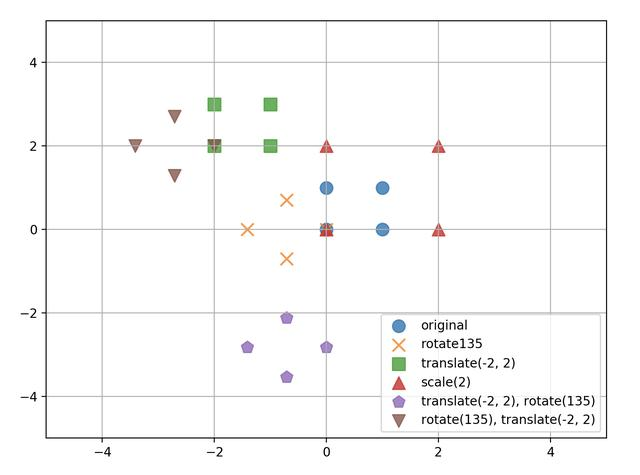需要注意的是，除少数例外情况外，矩阵通常不进行交换。即A1 @ A2 != A2 @ A1因此，对于变换# 平移然后旋转coords_composite1 = R1 @ T1 @ coords# 旋转然后平移coords_composite2 = T1 @ R1 @ coords你将看到它们不会产生相同的映射，而且顺序很重要。从右到左可以理解函数是如何应用的。Numpy中的变换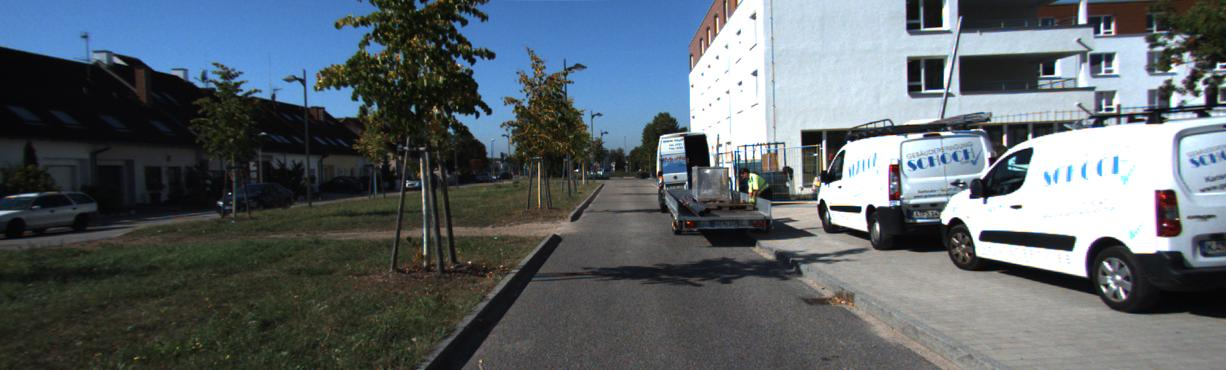现在对于图片，有几点需要注意。首先，如前所述，我们必须重新调整垂直轴。其次，变换后的点必须投影到图像平面上。实质上，需要采取的步骤是：创建新图像I'(x，y)以输出变换应用变换将点投影到新的图像平面上，仅考虑位于图像边界内的点。示例：围绕图像中心旋转、缩放和平移让我们看一个变换，我们希望放大2倍，并围绕图像的中心位置旋转45度。这可以通过应用以下复合矩阵来实现。height, width = image.shape[:2]tx, ty = np.array((width // 2, height // 2))angle = np.radians(45)scale = 2.0R = np.array([    [np.cos(angle), np.sin(angle), 0],    [-np.sin(angle), np.cos(angle), 0],    [0, 0, 1]])T = np.array([    [1, 0, tx],    [0, 1, ty],    [0, 0, 1]])S = np.array([    [scale, 0, 0],    [0, scale, 0],    [0, 0, 1]])A = T @ R @ S @ np.linalg.inv(T)应用于图像# 表示图像坐标的网格coords = get_grid(width, height, True)x_ori, y_ori = coords, coords # 应用变换warp_coords = np.round(A@coords).astype(np.int)xcoord2, ycoord2 = warp_coords[0, :], warp_coords[1, :]# 获取图像边界内的像素indices = np.where((xcoord >= 0) & (xcoord < width) &                   (ycoord >= 0) & (ycoord < height))xpix2, ypix2 = xcoord2[indices], ycoord2[indices]xpix, ypix = x_ori[indices], y_ori[indices]# 将像素RGB数据映射到另一个数组中的新位置canvas = np.zeros_like(image)canvas[ypix, xpix] = image[yy, xx]在上面的两个代码片段中有几点需要注意。左手坐标系旋转是通过交换符号来实现的。由于点围绕原点旋转，我们首先将中心平移到原点，然后再进行旋转和缩放然后将点变换回图像平面。将变换点舍入为整数以表示离散像素值。接下来，我们只考虑位于图像边界内的像素。映射对应的I(x，y)和I’(x，y)。如你所见，由于步骤4的原因，生成的图像将有几个锯齿和孔。为了消除这种情况，开源库使用插值技术来消除变换后的差异。逆扭曲(Inverse Warping)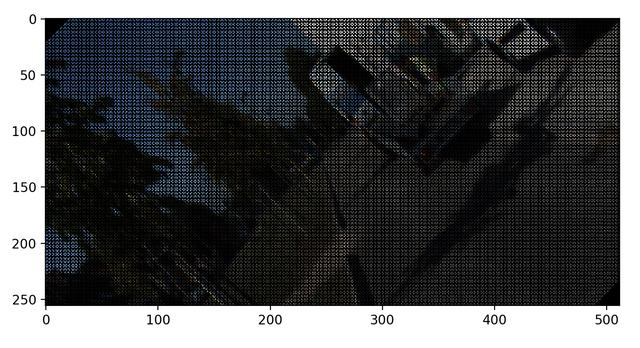另一种防止上面情况的方法是将扭曲表示为给定扭曲点x'的源图像I(x，y)的重采样。这可以通过X'乘以A的逆来实现。这里需要注意的是，变换必须是可逆的。将变换的逆运算应用到X'上。X = np.linalg.inv(A) @ X'注：对于图像，X'的逆扭曲只是将I'(X，y)重新投影到I(X，y)上。所以我们只需对I’(x，y)像素坐标进行逆变换，如下所示。确定它在原始图像平面中的位置对I(x，y)重新采样RGB像素并将其映射回I'(x，y)# 设置像素坐标I'(x，y)coords = get_grid(width, height, True)x2, y2 = coords, coords# 应用逆变换并舍入(最近邻插值)warp_coords = (Ainv@coords).astype(np.int)x1, y1 = warp_coords[0, :], warp_coords[1, :]# 获取图像边界内的像素indices = np.where((x1 >= 0) & (x1 < width) &                   (y1 >= 0) & (y1 < height))xpix1, ypix1 = x2[indices], y2[indices]xpix2, ypix2 = x1[indices], y1[indices]# 映射对应的像素canvas = np.zeros_like(image)canvas[ypix1, xpix1] = image[ypix2,xpix2]coords = get_grid(width, height, True)x2, y2 = coords, coords运行上面的代码应该可以得到一个密集的、无孔的图像。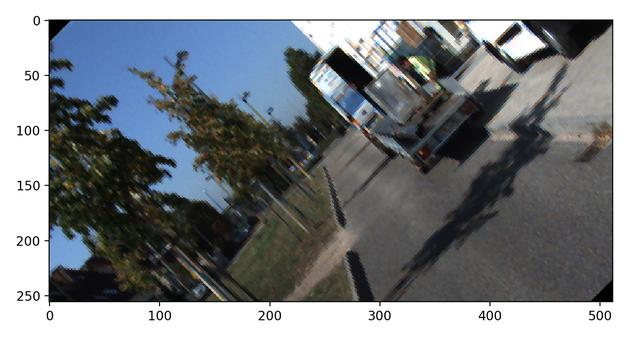OpenCV中的变换现在你已经对几何变换有了更好的理解，大多数开发人员和研究人员通常省去了编写所有这些变换的麻烦，而只需依赖优化的库来执行任务。在OpenCV中进行仿射变换非常简单。有几种方法可以做到。一种可能的方法是你可以自己编写仿射变换，并调用cv2.warfaffine(image，A，output_shape)下面的代码显示了整个仿射矩阵，它将给出与上面相同的结果。一个很好的练习就是自己推导公式！def get_affine_cv(t, r, s):    sin_theta = np.sin(r)    cos_theta = np.cos(r)        a_11 = s * cos_theta    a_21 = -s * sin_theta        a_12 = s * sin_theta    a_22 = s * cos_theta            a_13 = t * (1 - s * cos_theta) - s * sin_theta * t    a_23 = t * (1 - s * cos_theta) + s * sin_theta * t    return np.array([[a_11, a_12, a_13],                 [a_21, a_22, a_23]])A2 = get_affine_cv((tx, ty), angle, scale)warped = cv2.warpAffine(image, A2, (width, height))另一种方法是依赖OpenCV使用cv2.getRotationMatrix2D(center，angle，scale)返回仿射变换矩阵。此函数使用角度围绕点中心旋转图像，并使用比例缩放图像。A3 = cv2.getRotationMatrix2D((tx, ty), np.rad2deg(angle), scale)warped = cv2.warpAffine(image, b3, (width, height), flags=cv2.INTER_LINEAR, borderMode=cv2.BORDER_CONSTANT, borderValue=0)总结在本文中，我介绍了几何变换的基本概念以及如何将其应用于图像。许多先进的计算机视觉，如使用视觉里程计和多视图合成的slam，都依赖于最初的理解变换。我希望你能更好地理解这些公式是如何在库中编写和使用的。
展开全文• 实验内容 已知3×3的图像如图所示...定义一个a=zeros(300,300,3)三维矩阵，用for循环对该矩阵中的每个像素点进行赋值运算，给出不同值作为该图像每个像素点灰度级，生成的图像如图所示： 1.2将此图以图形中心...
• 1、确定旋转后新图像的尺寸，设原始大小为M,N 由以上公式得到： x=[0,(M-1)*cos(Angle),(M-1)*cos(Angle)+(N-1)*sin(Angle),(N-1)*sin(Angle)]; %旋转后新坐标系横坐标 y=[0,-(M-1)*sin(Angle),-(M-1)*sin(Angle)+...
• 文章目录图像的几何变换1 图像平移1.1 图像平移的变换公式1.2 MATLAB实现2 图像镜像2.1 图像镜像的变换公式2.2 MATLAB编程实现3 图像转置3.1 图像转置的变换公式3.2 MATLAB编程实现4 图像缩放4.1 图像缩放的变换公式...
• 第四节 几何变换图像生成过程，由于系统本身具有非线性或拍摄角度不同，会使生成的图像产生几何失真。几何失真一般分为系统失真和非系统失真，系统失真是有规律、能预测；非系统失真则是随机。例如：(1)镜头...
• % 图像缩放变换 双线性插值 % 输入： % img 灰白图像（彩色要多一个color维度或转化为灰度图） % s_x x方向上比例系数 % s_y y方向上比例系数 % 输出： % 缩放后图像 %% 双线性插值注意点： % 双线性插值...
• 2、（天坑）在matlab中，矩阵的行数是图像的高，矩阵的列数是图像的宽。在代码实现的过程中，尽量避免使用(i,j)/(x,y)，防止一不留神就拐进C++。（-,-） 我使用的是(rows,columns)，其中columns代表的是列数，即我们...计算机视觉
• 8.2 图像的几何运算本节介绍一种图像的基本变换，即几何变换。它主要是改变图像物体(像素)之间的空间关系，可以看成将各像素在图像内移动的过程。几何变换通常包括图像的平移、图像的镜像变换、图像的转置、图像的...
• 图像几何变换又叫做图像空间变换，它将一幅图像中的坐标位置映射到另一幅图像中的新坐标位置。关键：确定空间映射关系！ 1、图像平移 2、图像镜像 3、图像转置 4、图像缩放 5、图像旋转 　上述变换用到...
• 点击蓝字关注我们图像运算是图像处理常用的处理方法，它以图像为单位进行操作，运算的结果是一副新的图像，常常用于图像的高级处理(如图像分割，目标的检测和识别等)的前期处理。具体的图像运算包括点运算，代数...
• 本示例说明如何指定imwarp执行几何变换时使用填充值。执行转换时，输出图像中通常会有一些像素不属于原始输入图像。必须为这些像素分配一些值，称为填充值。默认情况下，imwarp将这些像素设置为零，并显示为黑色。...
• matlab的命令窗口输入doc imresize或者help imresize即可获得该函数帮助信息 调用格式 B = imresize(A, m) 返回的图像B长宽是图像A长宽m倍，即缩放图像。 m大于1，则放大图像; m小于1，缩小图像。 B...
• 8.2 图像的几何运算本节介绍一种图像的基本变换，即几何变换。它主要是改变图像物体(像素)之间的空间关系，可以看成将各像素在图像内移动的过程。几何变换通常包括图像的平移、图像的镜像变换、图像的转置、图像的...
• 几何变换之放大缩小 概念 1.缩小：从图像中，删除部分像素； 2. 放大：向图像中增加部分像素，增加像素取值是根据周围相邻像素值进行插值计算得到。 用法 调用格式： B = imresize(A,m) B = imresize(A,m,...
• 假设图像x轴方向缩放比率Sx，y轴方向缩放比率Sy，相应的变换表达式为：　其逆运算如下：　直接根据缩放公式计算得到目标图像中，某些映射源坐标可能不是整数，从而找不到对应像素位置。例如，当Sx=Sy=2时，...
• 图像的几何运算是指引起图像几何形状发生改变的变换。与点运算不同的是，几何运算可以看成是像素在图像内的移动过程，该移动过程可以改变图像物体对象之间的空间关系。 二、源代码 function varargout = aaa...
• 第2章 图像几何操作 2.1 图像的裁剪缩放与旋转 2.2 图像的几何变换 2.3 图像的邻域操作 2.4 图像的区域选取 2.5 图像增强 2.6 图像滤波 2.1 图像的裁剪缩放与旋转 图像裁剪 就是在原图像裁剪出图像块来 根据裁剪...
• 数字图像处理第五章 几何变换与图像配准几何变换与图像配准1 点变换2 仿射变换3 投影变换4 应用于图像的几何变换5 MATLAB中的图像坐标系统5 图像内插 几何变换与图像配准 几何变换改变了图像中像素间的空间关系，...
• ## MATLAB图像变换

千次阅读 2017-04-17 19:42:58
图像变换可以分为正交变换（积分变换）和几何变换两种。积分变换主要有离散傅里叶变换、离散余弦变换、小波变换等，其基本思想就是将图像信号从空间域变换到频率域。为什么要这样处理呢？目的是简化问题求解。举个...
• 也就是转换成唯一标准形式以抵抗仿射变换图像归一化使得图像可以抵抗几何变换的攻击，它能够找出图像中的那些不变量，从而得知这些图像原本就是一样或者一个系列。因为我们这次图片有好多都是一个系列，...
• 本示例通过将不同变换应用于棋盘图像来显示几何变换的许多属性。概览二维几何变换是将欧几里得平面中的每个点与欧几里得平面中的另一个点相关联映射。在这些示例几何变换由一条规则定义，该规则告诉如何将...
• 目录基本元素图片摄像头按位运算图像几何变换缩放：翻转：平移：旋转：透视变换基本元素图片 OpenCV彩色图是以B-G-R通道顺序存储，灰度图只有一个通道，图像坐标起始点是在左上角，所以行对应是y，列对应...opencv镜像
• 数字图像处理之几何变换（2）（一）Matlab中的图像坐标系统（1）输出图像位置（2）控制输出网格（二）图像内插（1）二维内插（2）内插方法比较 （一）Matlab中的图像坐标系统 在考虑几何变换的其他情况之前，......

# matlab中图像的几何变换matlab 订阅